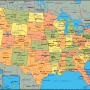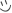# How well do you know the U.S. states and capitals?If you were educated in the United States, you probably had to memorize the states and capitals at some point. It's something that teachers like to do to mentally torture their students for no reason.

To make matters worse, the biggest or most prominent city in a state often actually isn't the capital city. How much do you remember? Give this quiz a try and see how you do!

Created by: Hiccstrid of tumblr

1. What is the capital of Alabama?
2. What is the capital of Alaska?
3. What is the capital of Arizona?
4. What is the capital of Arkansas?
5. What is the capital of California?
6. What is the capital of Colorado?
7. What is the capital of Connecticut?
8. What is the capital of Delaware?
9. What is the capital of Florida?
10. What is the capital of Georgia?
11. What is the capital of Hawaii?
12. What is the capital of Idaho?
13. What is the capital of Illinois?
14. What is the capital of Indiana?
15. What is the capital of Iowa?
16. What is the capital of Kansas?
17. What is the capital of Kentucky?
18. What is the capital of Louisiana?
19. What is the capital of Maine?
20. What is the capital of Maryland?
21. What is the capital of Massachusetts?
22. What is the capital of Michigan?
23. What is the capital of Minnesota?
24. What is the capital of Mississippi?
25. What is the capital of Missouri? (or, as I like to pronounce it, Misery :P -- no offense if you live there, of course!)
26. What is the capital of Montana?
27. What is the capital of Nebraska?
28. What is the capital of Nevada?
29. What is the capital of New Hampshire?
30. What is the capital of New Jersey?
31. What is the capital of New Mexico?
32. What is the capital of New York?
33. What is the capital of North Carolina?
34. What is the capital of North Dakota?
35. What is the capital of Ohio?
36. What is the capital of Oklahoma?
37. What is the capital of Oregon?
38. What is the capital of Pennsylvania?
39. What is the capital of Rhode Island?
40. What is the capital of South Carolina?
41. What is the capital of South Dakota?
42. What is the capital of Tennessee?
43. What is the capital of Texas?
44. What is the capital of Utah?
45. What is the capital of Vermont?
46. What is the capital of Virginia?
47. What is the capital of Washington?
48. What is the capital of West Virginia?
49. What is the capital of Wisconsin?
50. What is the capital of Wyoming?

You're about to get your result. Then try our new sharing options.